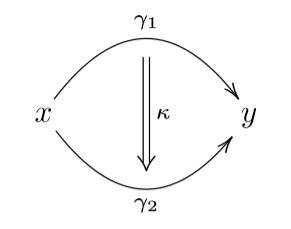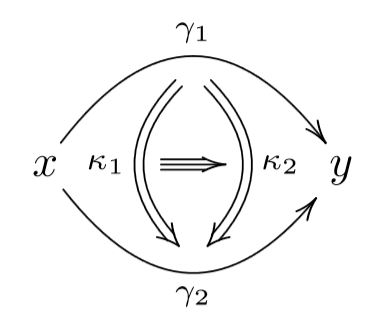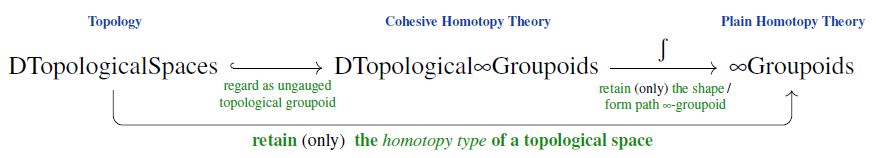Contents

# Contents

## Idea

### General idea

In generality, homotopy theory is the study of mathematical contexts in which functions or rather (homo-)morphisms are equipped with a concept of homotopy between them, hence with a concept of “equivalent deformations” of morphisms,and then iteratively with homotopies of homotopies between those:and so forth.

A key aspect here is that in such homotopy theoretic contexts the concept of isomorphism is relaxed to that of homotopy equivalence: Where a morphism is regarded as invertible if there is a reverse function such that both composites are equal to the identity morphism, for a homotopy equivalence one only requires the composites to be homotopic to the identity. Regarding objects in a homotopical context up to homotopy equivalence this way is to regard them as homotopy types.

It is not wrong to summarize this by saying that:

Homotopy theory embodies the gauge principle in mathematics.

Namely, in physics, the gauge principle says that it is wrong to ask for any two things to be equal, instead one always has to ask whether there is a gauge transformation relating them, and then a gauge-of-gauge transformation relating these, etc. Similarly in homotopy theory it is wrong to ask whether any two objects are equal, instead one has to ask whether there is a homotopy equivalence between them and then higher homotopies between these, etc.

For more exposition on the general idea of homotopy theory see:

### Topological homotopy theoryThe archetypical example of a homotopy theory is the classical homotopy theory of topological spaces, where one considers topological spaces with continuous functions between them, and with the original concept of topological homotopies between these continuous functions.

The category whose objects are topological spaces and whose morphisms are homotopy equivalence-class of continuous functions is also called the classical homotopy category.

Classical constructions in topological homotopy theory are homotopy groups, Toda brackets, long exact sequences of homotopy groups, …

This classical homotopy theory of topological spaces has many applications, for example to covering space theory, to classifying space theory, to Whitehead-generalized cohomology theory and many more. (See also at shape theory.) Accordingly, homotopy theory has a large overlap with algebraic topology.

For more exposition of topological homotopy theory see:

### Abstract homotopy theory

The basic structures seen in classical homotopy theory of topological spaces may be abstracted to yield an “abstract homotopy theory” that applies to a large variety of contexts. There are several more or less equivalent formalizations of the concept of “abstract homotopy theory”, including

The terminology model category is short for “category of models of homotopy types”. The idea here is to consider categories equipped with suitable extra structure and properties that encodes the existence of homotopies between all morphisms and convenient means to handle and control these, in particular a means to construct the corresonding homotopy category.

For a detailed introduction to abstract homotopy theory via model cateories see:

The approach of (∞,1)-categories to homotopy theory is meant to be more truthful to the intrinsic nature of homotopy theory. Instead of equipping an ordinary category with a extra concept of homotopy between its morphisms, here one regards the resulting structure as a higher category where the homotopies themselves appear as a kind of higher order morphisms, called 2-morphisms and where higher homotopies of homotopies are regarded as k-morphisms for all $k$.

The terminology “(∞,1)-category” signifies that homotopy theory is but one special case of general higher category theory, namely (∞,1)-categories (hence homotopy theories) are those infinity-categories in which all k-morphisms for $k \gt 1$ are invertible up to homotopy. If one drops this constraint, so that homotopies become “directed” then one might still speak of “directed homotopy theory”.

The archetypical example of an (∞,1)-category is the $(\infty,1)$-category ∞Grpd of ∞-groupoids, just as Set is the archetypical 1-category.

This turns out to be equivalent, as homotopy theories, to the classical homotopy theory of topological spaces if restricted to those that admit the structure of CW-complexes.

### Relation between topological and abstract homotopy theory: Cohesion.

The case of the homotopy theory of topological spaces (above) is so classical that often the distinction between topology and homotopy theory has been (certainly so in the early literature) and still is often blurred (for instance in textbook titles or lecture notes). A transparent conceptual way to understand how topology and homotopy theory are closely related and yet different is provided by the notion of cohesion:

A topological space $X$ has (or presents) a homotopy type through the data that is retained in its path ∞-groupoid, conveniently modeled by its singular simplicial set $Sing(X)$ (which is a Kan complex and as such an ∞-groupoid). But the topological space contains more information than that retained in its path ∞-groupoid – the latter is just a shadow of it, called the shape of the topological space.

The extra information in a topological space is the cohesion on its underlying set of points: The geometric information of how these points stick together within open subsets. This information is lost when one regards topological spaces as stand-ins for their shapes (path ∞-groupoids) in plain homotopy theory.

More formally:

The category of D-topological spaces is a full subcategory of the cohesive $(\infty,1)$-topos of D-topological $\infty$-groupoids (also of smooth $\infty$-groupoids). These carry a qualitative aspect called their shape, which is a generalization of the fundamental ∞-groupoid-construction. Now, regarding a topological space as an object in (plain) homotopy theory, as traditionally done, really means to first regard it as a topological groupoid in cohesive homotopy theory and then, as such, to retain only its shape. The result is the homotopy type which is “presented” by the topological space:### Simplicial homotopy theory

The most immediate way model an ∞-groupoid is as a simplicial set which is a Kan complex. Accordingly, another homotopy theory equivalent to archetypical homotopy theory of ∞-groupoids is the classical homotopy theory of simplicial sets, typically referred to as simplicial homotopy theory.

### Flavors of homotopy theory

But there are many other homotopy theories besides (the various incarnations of) this classical one. Important sub-classes of homotopy theories include:

The geometric homotopy theory of (∞,1)-toposes in particular serves as the foundation for higher geometry/derived geometry. This is relevant notably in the physics of gauge theory, where gauge transformations are identified with homotopies in geometric homotopy theory. For more on this see at geometry of physics – homotopy types.

On the other hand, the incarnation of homotopy theory as homotopy type theory exhibits the remarkably foundational nature of homotopy theory. Contrary to its original appearance as a fairly complicated-looking theory built on top of classical set theory and classical topology, homotopy theory turns out to be intrinsically simple: it arises from plain dependent type theory just by adopting a fully constructive attitude towards the concept of identity/equality, see at identity type for more on this. For exposition of this perspective see (Shulman 17).

## Presentations

A convenient, powerful, and traditional way to deal with (∞,1)-categories is to “present” them by 1-categories with specified classes of morphisms called weak equivalences : a category with weak equivalences or homotopical category. The idea is as follows. Given a category $C$ with a class $W$ of weak equivalences, we can form its homotopy category or category of fractions $C[W^{-1}]$ by adjoining formal inverses to all the morphisms in $W$. The (∞,1)-category presented by $(C,W)$“ can be thought of as the result of regarding $C$ as an $\infty$-category with only identity $k$-cells for $k\gt 1$, then adjoining formal inverses to morphisms in $W$ in the $\infty$-categorical sense; that is, making them into equivalences rather than isomorphisms. It is remarkable that most $(\infty,1)$-categories that arise in mathematics can be presented in this way.

As with presentations of groups and other algebraic structures, very different presentations can give rise to equivalent $(\infty,1)$-categories. For example, several different presentations of the $(\infty,1)$-category of $\infty$-groupoids are:

The latter three can hence be regarded as providing “combinatorial models” for the homotopy theory of topological spaces.

### Model Categories

The value of working with presentations of $(\infty,1)$-categories rather than the $(\infty,1)$-categories themselves is that the presentations are ordinary 1-categories, and thus much simpler to work with. For instance, ordinary limits and colimits are easy to construct in the category of topological spaces, or of simplicial sets, and we can then use these to get a handle on $(\infty,1)$-categorical limits and colimits in the $(\infty,1)$-category of $\infty$-groupoids. However, we always have to make sure that we use only 1-categorical constructions that are homotopically meaningful, which essentially means that they induce $(\infty,1)$-categorical meaningful constructions in the presented $(\infty,1)$-category. In particular, they must be invariant under weak equivalence.

Most presentations of $(\infty,1)$-categories come with additional classes of morphisms, called fibrations and cofibrations, that are very useful in performing constructions in a homotopically meaningful way. Quillen defined a model category to be a 1-category together with classes of morphisms called weak equivalences, cofibrations, and fibrations that fit together in a very precise way (the term is meant to suggest “a category of models for a homotopy theory”). Many, perhaps most, presentations of $(\infty,1)$-categories are model categories. Moreover, even when we do not have a model category, we often have classes of cofibrations and fibrations with many of the properties possessed by cofibrations and fibrations in a model category, and even when we do have a model category, there may be classes of cofibrations and fibrations, different from those in the model structure, that are useful for some purposes.

Unlike the weak equivalences, which determine the “homotopy theory” and the $(\infty,1)$-category that it presents, fibrations and cofibrations should be regarded as technical tools which make working directly with the presentation easier (or possible). Whether a morphism is a fibration or cofibration has no meaning after we pass to the presented $(\infty,1)$-category. In fact, every morphism is weakly equivalent to a fibration and to a cofibration. In particular, despite the common use of double-headed arrows for fibrations and hooked arrows for cofibrations, they do not correspond to $(\infty,1)$-categorical epimorphisms and monomorphisms.

In a model category, a morphism which is both a fibration and a weak equivalence is called an acyclic fibration or a trivial fibration. Dually we have acyclic or trivial cofibrations. An object $X$ is called cofibrant if the map $0\to X$ from the initial object to $X$ is a cofibration, and fibrant if the map $X\to 1$ to the terminal# object is a fibration. The axioms of a model category ensure that for every object $X$ there is an acyclic fibration $Q X \to X$ where $Q X$ is cofibrant and an acyclic cofibration $X\to R X$ where $R X$ is fibrant.

### Examples

For a (higher) category theorist, the following examples of model categories are perhaps the most useful to keep in mind:

• $C=$ sets, $W=$ isomorphisms. All morphisms are both fibrations and cofibrations. The $(\infty,1)$-category presented is again the 1-category $Set$.
• $C=$ categories, $W=$ equivalences of categories. The cofibrations are the functors which are injective on objects, and the fibrations are the isofibrations. The acyclic fibrations are the equivalences of categories which are literally surjective on objects. Every object is both fibrant and cofibrant. The $(\infty,1)$-category presented is the 2-category $Cat$. This is often called the folk model structure.
• $C=$ (strict) 2-categories and (strict) 2-functors, $W=$ 2-functors which are equivalences of bicategories. The fibrations are the 2-functors which are isofibrations on hom-categories and have an equivalence-lifting property. Every object is fibrant; the cofibrant 2-categories are those whose underlying 1-category is freely generated by some directed graph. The $(\infty,1)$-category presented is the (weak) 3-category $2Cat$. This model structure is due to Steve Lack.

### Generalized Morphisms

The morphisms from $A$ to $B$ in the $(\infty,1)$-category presented by $(C,W)$ are zigzags $\stackrel{\simeq}{\leftarrow} \to \stackrel{\simeq}{\leftarrow} \to \cdots$; these are sometimes called generalized morphisms. Many presentations (including every model category) have the property that any such morphism is equivalent to one with a single zag, as in $\stackrel{\simeq}{\leftarrow} \to \stackrel{\simeq}{\leftarrow}$. In a model category, a canonical form for such a zigzag is $X \stackrel{\simeq}{\leftarrow} Q X \to R Y \stackrel{\simeq}{\leftarrow} Y$ where $Q X$ is cofibrant and $R Y$ is fibrant. In this case we can moreover take $Q X\to X$ to be an acyclic fibration and $Y\to R Y$ to be an acyclic cofibration.

Often it suffices to consider even shorter zigzags of the form $\stackrel{\simeq}{\leftarrow} \to$ or $\to \stackrel{\simeq}{\leftarrow}$. In particular, this is the case if every object is fibrant or every object is cofibrant. For example:

• If $X$ and $Y$ are strict 2-categories, then pseudofunctors $X\to Y$ are equivalent to strict 2-functors $Q X \to Y$, where $Q X$ is a cofibrant replacement for $X$.
• anafunctors are zigzags $\stackrel{\simeq}{\leftarrow} \to$ in the folk model structure on 1-categories whose first factor is an acyclic (i.e. surjective) fibration.
• Morita morphisms in the theory of Lie groupoids are generalized morphisms of length one where both maps are acyclic fibrations.

If $X$ is cofibrant and $Y$ is fibrant, then every generalized morphism from $X$ to $Y$ is equivalent to an ordinary morphism. For example, if $X$ is a cofibrant 2-category, then every pseudofunctor $X\to Y$ is equivalent to a strict 2-functor $X\to Y$

### Quillen Equivalences

Quillen also introduced a highly structured notion of equivalence between model categories, now called a Quillen equivalence, which among other things ensures that they present the same $(\infty,1)$-category. Quillen equivalences are now being used to compare different definitions of higher categories.

## Related entries

### Categorical homotopy theory

(…)

The following lists basic references on homotopy theory, algebraic topology and some $(\infty,1)$-category theory and homotopy type theory, but see these entries for more pointers.

### Pre-history

Historical article at the origin of all these subjects:

On early developments from there, such as the eventual understanding of the notion of higher homotopy groups:

• Peter Hilton, Subjective History of Homology and Homotopy Theory, Mathematics Magazine 61 5 (1988) 282-291 $[$doi:10.2307/2689545$]$

### Topological homotopy theory

Textbook accounts of homotopy theory of topological spaces (i.e. via “point-set topology”):

### Algebraic topology

Textbooks:

On constructive methods (constructive algebraic topology):

Lecture notes:

Survey of various subjects in algebraic topology:

Survey with relation to differential topology:

Some interactive 3D demos:

Further pointers:

### Abstract homotopy theory

On localization via model category-theory:

On localization (especially of categories of simplicial sheaves/simplicial presheaves) via categories of fibrant objects:

Lecture notes:

Introduction, from category theory to (mostly abstract, simplicial) homotopy theory:

### Basic homotopy type theory

Exposition:

Textbook accounts:

For more see also at homotopy theory formalized in homotopy type theory.

### Outlook

Indications of open questions and possible future directions in algebraic topology and (stable) homotopy theory:

More regarding the sociology of the field (such as its folklore results):